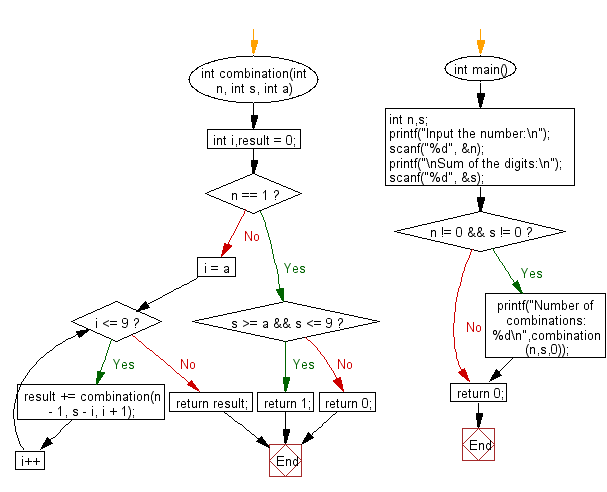﻿ C Program: Sum of the digits equals to another given number - w3resource

# C Exercises: Sum of the digits equals to another given number

## C Basic Declarations and Expressions: Exercise-141 with Solution

Write a C program that reads n digits (given) chosen from 0 to 9 and prints the number of combinations where the sum of the digits equals another given number (s). Do not use the same digits in a combination.

For example, the combinations where n = 3 and s = 6 are as follows:
1 + 2 + 3 = 6
0 + 1 + 5 = 6
0 + 2 + 4 = 6

Sample Solution:

C Code:

``````#include<stdio.h>

// Recursive function to find combinations
int combination(int n, int s, int a) {
int i, result = 0;

// Base case: if n is 1, check if s is within valid range
if (n == 1) {
if (s >= a && s <= 9) {
return 1;
} else {
return 0;
}
}

// Iterate through possible values (a to 9)
for (i = a; i <= 9; i++) {
// Recursively call combination for (n-1) and adjust s and a values
result += combination(n - 1, s - i, i + 1);
}

return result; // Return the total combinations
}

int main() {
int n, s;

printf("Input the number:\n");
scanf("%d", &n);
printf("\nSum of the digits:\n");
scanf("%d", &s);

if (n != 0 && s != 0) {
printf("Number of combinations: %d\n", combination(n, s, 0));
}

return 0;
}
``````

Sample Output:

```Input the number:
3

Sum of the digits:
6
Number of combinations: 3
```

Flowchart:C programming Code Editor:

What is the difficulty level of this exercise?

Test your Programming skills with w3resource's quiz.

﻿